# Physics Test 10 - Heat And Thermodynamics

## 25 Questions MCQ Test Mock Test Series for JEE Main & Advanced 2022 | Physics Test 10 - Heat And Thermodynamics

Description
Attempt Physics Test 10 - Heat And Thermodynamics | 25 questions in 60 minutes | Mock test for JEE preparation | Free important questions MCQ to study Mock Test Series for JEE Main & Advanced 2022 for JEE Exam | Download free PDF with solutions
QUESTION: 1

### For Boyle’s law to hold good, the gas should be

Solution: For Boyle's law gases must obey ideal gas equation
PV=nRT
Boyles law states that for a fixed amount of gas at constant temperature pressure is inversely proportional to its volume.i.e.PV=constant
QUESTION: 2

### A bulb contains 1 mole of hydrogen and 1 mole of oxygen at temperature T. The ratio of rms values of velocity of hydrogen molecules to that of oxygen molecule is

Solution: RMS speed sqroot of 3RT/M M=molar mass T=temp R=cont used it
QUESTION: 3

### An ideal gas is heated from 270 to 6270 C at constant pressure. If initial volume was 4m3,then the final volume of gas will be

Solution:
QUESTION: 4

Critical temperature can be difined as the temperature

Solution:
QUESTION: 5

A molecule of mass m of an ideal gas collides with the wall of the vessel with the same velocity. The change in the linear momentum of the molecule will be

Solution:
Change in linear momentum = Final
Momentum-Initial momentum.
Momentum = mass x velocity
Initial velocity = v
Since molecule returns back with same velocity, final velocity = -v
Initial momentum = mv
Final momentum = -mv
Change in linear momentum is = -mv-(mv)=-2mv
QUESTION: 6

Rate of diffusion is

Solution:
QUESTION: 7

Which one of the following is not a thermodynamical co-ordinate ?

Solution:
QUESTION: 8

The mean kinetic energy of a molecule of a gas at 300 K is 6.21 x 10-21 The root mean square velocity of a molecule of H2 gas at this temperature will be(R=8.3 J/mole K,N= 6.02 x 1023 /mole, mass of the
hydrogen moleucle = 2 x 1.67 x 10-27 Kg)

Solution:
QUESTION: 9

At what temperautre will the rms velocity of oxygen moleucle be sufficient, so as to escape from the earth ? Escape velocity from the earth is 11.0 km/s and the mass of 1 moleucle of oxygen 5.34 x 10-26 (Boltzman constant k =1.38 x 10-23 J/K)

Solution:
QUESTION: 10

The temperature of inside and outside of a refrigerator are 273 K and 303 K respectively.  Assuming that the regrigerator cycle is reverisble,for every joule of work done, the heat delivered to the surrounding will be nearly

Solution: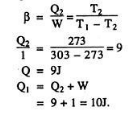QUESTION: 11

A sample of gas expands from volume V1 to V2. The amount of work done by the gas is greatest, when the expansion is

Solution: While expanding the PV diagram shows drop in pressure for isothermal and even more in adiabatic process, but in isobaric process pressure remains constant ie parallel to V axis. the work done in P V diagram is area under PV curve between the limits of volumes. hence the area is maximum for isobaric then comes isothermal and adiabatic has the least work done for a given range of volumes.
QUESTION: 12

During the adiabatic expansion of 2 moles of a gas the internal energy of a gas is found to decrease by 2 J. The work done during the process on gas will be equal to

Solution: In any adiabatic process heat is not given or taken out of system , so there will be adiabatic expansion it will be on expense of its own internal energy so we see decrease in internal energy by first law of thermodynamics that when heat is given to a system it either is used in doing work or increasing internal energy,  when no heat is given and system is expanding it has to spend it's own internal energy appearing as work done.
QUESTION: 13

Magnitude of slope of an adiabatic curve is always

Solution:
QUESTION: 14

Air is filled in a bottle at atmoshperic pressure and it is cordked at 350C. If the cork can come out at 3 atmospheric pressure then upto what temperature should the bottle be heated in order to remove the cork?

Solution:
P1 V1 / T1 = P2 V2 / T2
Volume is constant (bottle volume). So,
P1 / T1 = P2 / T2
1 / (273+35) = 3 / T2
So temperature to be heated is,
651 C.
At which bottle would have vaporized.
QUESTION: 15

A perfect gas goes from state A to another state B by absorbing  8 x 105 J of heat and doing 6.5 x 105 J  of expernal work. It is now transfered between the same two states in another process in which it absorbs 105 J of heat. Then, in the second process

Solution: Now ΔQ=ΔW +ΔE

For first case, 8 x 10'5=6.5 x 10'5+ΔE

Therefore, ΔE=1.5 x 10'5 J

In the second case, ΔQ=10'5 J

Therefore ΔW=ΔQ-ΔE

=-0.5 x 10'5 so answer option A correct
QUESTION: 16

If 150 J of heat is added to a system and the work done by the system is 110 J. Then, change in internal energy will be

Solution:
QUESTION: 17

An ideal refirgator has a freezer at a temperature of -130C. The coefficient of performance of the engine is 5. The temperature of the air (to which heat is rejected) will be

Solution:
QUESTION: 18

A Carnot reversible engine converts 1/6 of heat input work. When the temperature of the sink is reduced by 62 K, the efficiency of Carnot’s cycle becomes 1/3. The temperature of the source and sink will be

Solution:
QUESTION: 19

When a solid is converted into a gas,directly by heating then this process is known as

Solution:
QUESTION: 20

The latent heat of vapourization of water is 2240 J.If the work done in the process of vapourization of 1 g is 168 J, then increase in internal energy will be

Solution:
*Answer can only contain numeric values
QUESTION: 21

A vessel contains a mixture of 7 g nitrogen and 11 g of carbondioxide at temperature T = 290K. If the pressure of the mixture is 1 atm (1.01 × 105 N/m2). Calculate its density in kg/m3:-

Solution:
*Answer can only contain numeric values
QUESTION: 22

To form a composite 16 μF, 1000V capacitor from a supply of identical capacitors marked 8 μF, 250 V, we require a minimum number of capacitors.

Solution:
*Answer can only contain numeric values
QUESTION: 23

If the height of transmitting and receiving antenna are 32 m and 50 m respectively, then for line of sight (LOS) propagation maximum distance between both antenna is (If radius of earth = 6400 km) (in km) :-

Solution:
*Answer can only contain numeric values
QUESTION: 24

Figure, shows a circuit in which three identical diodes are used. Each diode has forward resistance of 20Ω and infinite backward resistance. Resistors R1 = R2 = R3 = 50 Ω. Battery voltage is 6V. Find the value of current in mA through R3?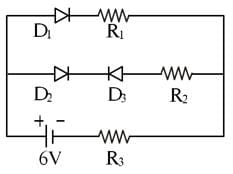Solution:

Since D3 is reverse biased, there is not current through that branch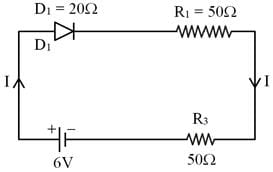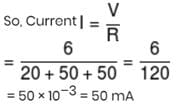*Answer can only contain numeric values
QUESTION: 25

A horizontal pipe has a cross section of 10 cm2 in one region and of 5 cm2 in another. The water velocity at the first is 5 ms–1 and the pressure in the second is 2 × 105 Nm–2. Find the pressure of water in 1st region (in N/m2).

Solution: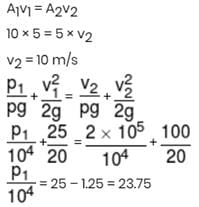Use Code STAYHOME200 and get INR 200 additional OFF Use Coupon Code